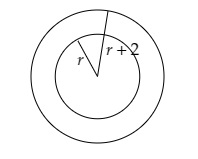# The area of a circle is $616 \mathrm{~cm}^{2}$. There is a $2 \mathrm{~m}$ wide road on its edge. What will be area of that road?

Given:

The area of a circle is $616 \mathrm{~cm}^{2}$. There is a $2 \mathrm{~m}$ wide road on its edge.

To do:

We have to find the area of the road.
Solution:

Let the radius of the inner circle be $r$.Width of the road $=2\ m$.

This implies,

Radius of the outer circle$=r+2\ m$.
Area of the inner circle$=616\ m^2$

Therefore,

$\pi r^2=616$

$\frac{22}{7}\times r^2=616$

$r^2=616\times\frac{7}{22}$

$r^2=28\times7$

$r^2=7\times4\times7$

$r^2=(2\times7)^2$

$r=14\ m$

Radius of the outer circle$=14+2\ m=16\ m$.

Area of the outer circle$=\pi (16)^2$

$=\frac{22}{7}\times256$

$=804.57\ m^2$

Area of the road$=$Area of the outer circle$-$ Area of the inner circle

$=804.57-616\ m^2$

$=188.57\ m^2$

The area of the road is $188.57\ m^2$.

Updated on: 10-Oct-2022

90 Views